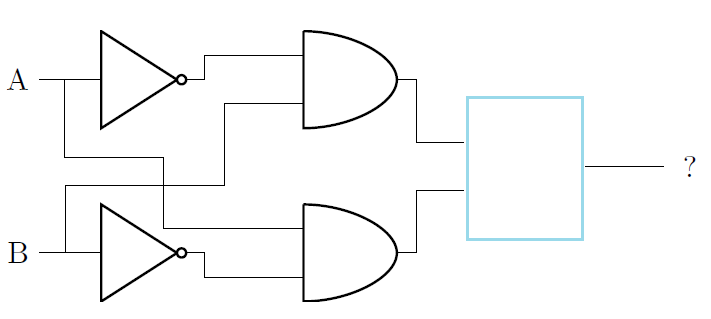# Propositional Logic: Logic Gates Intermediate Warmup

Logic Level 1The setup above works like a XOR gate (the output will be 1 if exactly one of the inputs is 1). However, the gate marked by a blue box is missing. What is the gate?

×